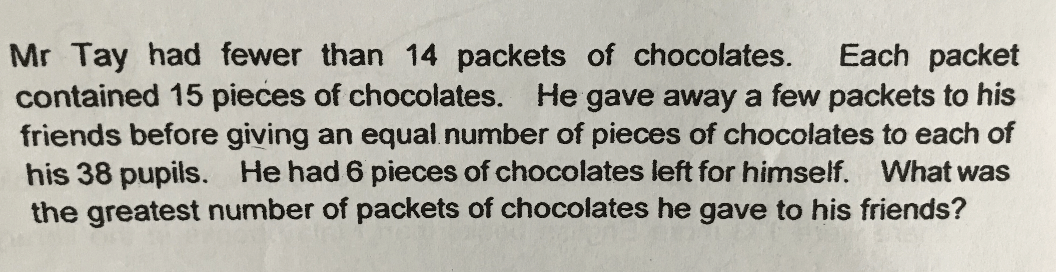# QuestionSource: Nanyang Primary

1 Answer

# Answer

6 + 38 = 44

44, 82, 120, …
120/15 = 8
(14 – 1) – 8 = 5

Ans : 5 packets.

1 Reply 0 Likes

Thanks. May I ask why 44, 82,120?

1 Reply 0 Likes

6 + 38 = 44

44 + 38 = 82

82 + 38 = 120

0 Replies 1 Like